# 20183413 2019-2020-2 《Python程序设计》实验二报告

## 1.实验内容

1.设计一个简单的计算器，内容包括：

• 简单的四则运算（可同时包含多个加减乘除运算，但不支持含括号的运算）
• 求模运算
• 求阶乘
• 指数运算
• 角度和弧度的转化
• 基本三角函数

2.练习条件、循环等基本语句以及函数、类等知识点。

## 2.实验过程及实验结果

### 四则运算

class basic:
'''
基本四则运算（无法进行带括号的运算）
'''
def __init__(self, expression):
self.expression = expression
​
def addition(self, expression): #加法
num = expression.split('+',1)
return float(num) + float(num)
​
def subtraction(self, expression): #减法
if expression.startswith('-') :#第一个数字是负数
expression = expression[1:]
num = expression.split('-',1)
num = '-' + num
else :
num = expression.split('-',1)
return float(num) - float(num)
​
def multiplication(self, expression): #乘法
num = expression.split('*',1)
return float(num) * float(num)
​
def devision(self, expression): #除法
num = expression.split('/',1)
return float(num) / float(num)
​
def calculation(self, expression): #判断运算式为何种运算
expression = expression.group()
if '+' in expression:
return self.addition(expression)
if '-' in expression:
return self.subtraction(expression)
if '*' in expression:
return self.multiplication(expression)
if '/' in expression:
return self.devision(expression)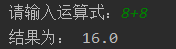def sorting(self, expression): #按运算顺序计算
pattern_high =  re.compile('(\d+\.?\d*(\*|\/)+\-?\d+\.?\d*)') #高运算级
pattern_low = re.compile('(\-?\d+\.?\d*(\+|\-)+\d+\.?\d*)') #低运算级
if re.search(pattern_high, expression): #递归
expression = re.sub(pattern_high, self.calculation, expression)
return self.sorting(expression)
elif re.search(pattern_low, expression):
expression = re.sub(pattern_low, self.calculation, expression)
return self.sorting(expression)
else:
expression
return expression
expression = input('请输入运算式：')
count = basic(expression)
result = count.sorting(expression)
print('结果为：', result)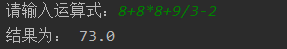### 求模运算与指数运算

class mod_function():
'''
求模运算
'''
def __init__(self,expression):
self.expression = expression
​
def calculation(self,expression):
num = expression.split('%')
return int(num) % int(num)
​
class index_function():
'''
指数运算
'''
def __init__(self,expression):
self.expression = expression
​
def calculation(self,expression):
num = expression.split('^')
return int(num) ** int(num)
​
​
expression = input('请输入计算式如：x % y\n')
mod = mod_function(expression)
result = mod.calculation(expression)
print('结果为：', result)
​
expression = input('请输入计算式如：x ^ y\n')
index = index_function(expression)
result = index.calculation(expression)
print('结果为：',result)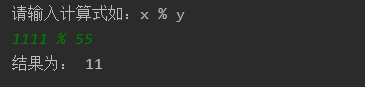### 求阶乘

z = int(input('请输入求阶乘的值：'))
result = 1
for i in range(1,z + 1):
result = result * i
print('结果为：',result)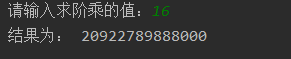### 弧度/角度转换

degree = float(input('输入角度：'))
print('结果为：',math.radians(degree))
​
​
rad = float(input('输入弧度：'))
print('结果为：',math.degrees(rad))### 简单三角函数

class degree_function():
'''
求三角函数（输入为角度）
'''
def __init__(self,expression):
self.expression = expression
​
def calculation(self,expression):
#expression = expression.group()
if 'sin' in expression:
num = expression.split('sin')
degree = float(num)
return math.sin(math.radians(degree))
elif 'cos' in expression:
num = expression.split('cos')
degree = float(num)
return math.cos(math.radians(degree))
elif 'tan' in expression:
num = expression.split('tan')
degree = float(num)
return math.tan(math.radians(degree))
​
​
expression = input('请输入计算式：')
degree_1 = degree_function(expression)
result = degree_1.calculation(expression)
print('结果为：',result)'''

'''

import re
import math

class basic:
'''
基本四则运算（无法进行带括号的运算）
'''
def __init__(self, expression):
self.expression = expression

def addition(self, expression): #加法
num = expression.split('+',1)
return str(float(num) + float(num))

def subtraction(self, expression): #减法
if expression.startswith('-') :#第一个数字是负数
expression = expression[1:]
num = expression.split('-',1)
num = '-' + num
else :
num = expression.split('-',1)
return str(float(num) - float(num))

def multiplication(self, expression): #乘法
num = expression.split('*',1)
return str(float(num) * float(num))

def devision(self, expression): #除法
num = expression.split('/',1)
return str(float(num) / float(num))

def calculation(self, expression): #判断运算式为何种运算
expression = expression.group()
if '+' in expression:
return self.addition(expression)
if '-' in expression:
return self.subtraction(expression)
if '*' in expression:
return self.multiplication(expression)
if '/' in expression:
return self.devision(expression)

def sorting(self, expression): #按运算顺序计算
pattern_high =  re.compile('(\d+\.?\d*(\*|\/)+\-?\d+\.?\d*)') #高运算级
pattern_low = re.compile('(\-?\d+\.?\d*(\+|\-)+\d+\.?\d*)') #低运算级
if re.search(pattern_high, expression): #递归
expression = re.sub(pattern_high, self.calculation, expression)
return self.sorting(expression)
elif re.search(pattern_low, expression):
expression = re.sub(pattern_low, self.calculation, expression)
return self.sorting(expression)
else:
expression
return expression

class mod_function():
'''
求模运算
'''
def __init__(self,expression):
self.expression = expression

def calculation(self,expression):
num = expression.split('%')
return int(num) % int(num)

class index_function():
'''
指数运算
'''
def __init__(self,expression):
self.expression = expression

def calculation(self,expression):
num = expression.split('^')
return int(num) ** int(num)

class degree_function():
'''
求三角函数（输入为角度）
'''
def __init__(self,expression):
self.expression = expression

def calculation(self,expression):
#expression = expression.group()
if 'sin' in expression:
num = expression.split('sin')
degree = float(num)
return math.sin(math.radians(degree))
elif 'cos' in expression:
num = expression.split('cos')
degree = float(num)
return math.cos(math.radians(degree))
elif 'tan' in expression:
num = expression.split('tan')
degree = float(num)
return math.tan(math.radians(degree))

menu='''
1--基本四则运算   2--求模运算   3--指数运算   4--求阶乘
5--角度转弧度   6--弧度转角度   7--三角函数(sin cos tan)
0.退出
'''

while(1):
print(menu)
a = input('请选择：')
if a == '1':
expression = input('请输入运算式：')
count = basic(expression)
result = count.sorting(expression)
print('结果为：', result)
elif a == '2':
expression = input('请输入计算式如：x % y\n')
mod = mod_function(expression)
result = mod.calculation(expression)
print('结果为：', result)
elif a == '3':
expression = input('请输入计算式如：x ^ y\n')
index = index_function(expression)
result = index.calculation(expression)
print('结果为：',result)
elif a == '4':
z = int(input('请输入求阶乘的值：'))
result = 1
for i in range(1,z + 1):
result = result * i
print('结果为：',result)
elif a == '5':
degree = float(input('输入角度：'))
print('结果为：',math.radians(degree),'rad')
elif a == '6':
rad = float(input('输入弧度：'))
print('结果为：',math.degrees(rad),'度')
elif a == '7':
expression = input('请输入计算式：')
degree_1 = degree_function(expression)
result = degree_1.calculation(expression)
print('结果为：',result)
elif a == '0':
break
else:
print('输入错误，请重新输入！')

## 3.实验过程中遇到的问题和解决方法

• 问题1：在最初设计四则运算时无法进行多个数的复杂运算

问题1解决方案：一开始打算把运算式中的所有运算符号都切割开，再使用if语句一个个判断，最后得出结果，但是这样很难实现，所以不得不放弃。后查阅资料发现可以使用正则表达式，于是自己尝试写了两个正则表达式提取优先级，最后再迭代运算，就能较好的解决问题。唯一的遗憾是是对含括号的运算束手无策。

• 问题2：在使用递归函数迭代运算时系统提示出错，无法得出结果

问题2解决方案：在调试程序后发现，迭代后原本切割后形成的两个字符串还要再进行切割，因此要将运算后得到的结果转化为str型，才能继续进行迭代，而不能直接返回float或者int型的结果。

• 问题3：一开始进行四则运算时函数太多，显得关系很乱

问题3解决方案：创建一个class类，将四则运算有关的函数写进类的方法里，这样一来调用函数就更加简便，逻辑更加清晰，后续的其他运算也采用此方法。

## 其他

• 在去年学C语言时我也做过设计计算器的实验，当时写的比较简单，而这次老师没有具体要求，所以我希望挑战一下更高难度的（虽然这对于其他大佬来说还是十分简单）。在使用 字符串相关函数对字符串进行切割、截取时，我体会到了python的简洁高效，一些看似不起眼的函数可以实现很强大的功能，这是我以前不敢现象的。在实验过程中我也遇到了不少困难，我买的教材上缺少正则表达式和字符串函数的部分内容，导致我在操作中走了不少弯路，最后通过上网查找才解决了一些问题。python是个巨大的宝藏，我现在认识到的还仅仅是冰山一角，以后还要不断练习、学习新的知识，温故知新，才能更好的掌握这个工具。

# 20183413 2019-2020-2 《Python程序设计》实验一报告

## 1.实验内容

• 熟悉Python开发环境；
• 练习Python运行、调试技能；
• 编写程序，练习变量和类型、字符串、对象、缩进和注释等；
• 掌握git技能

## 练习python运行及调试技能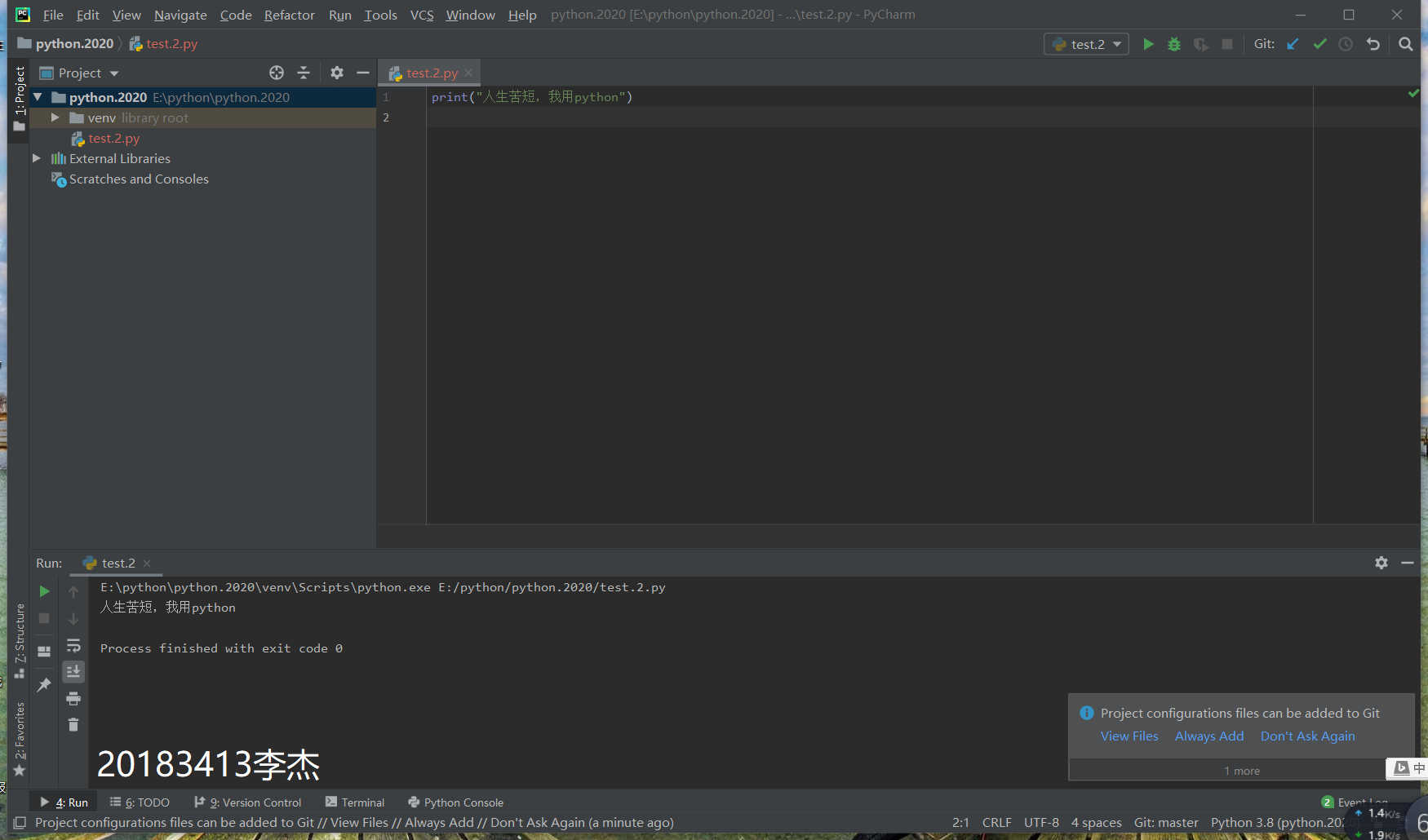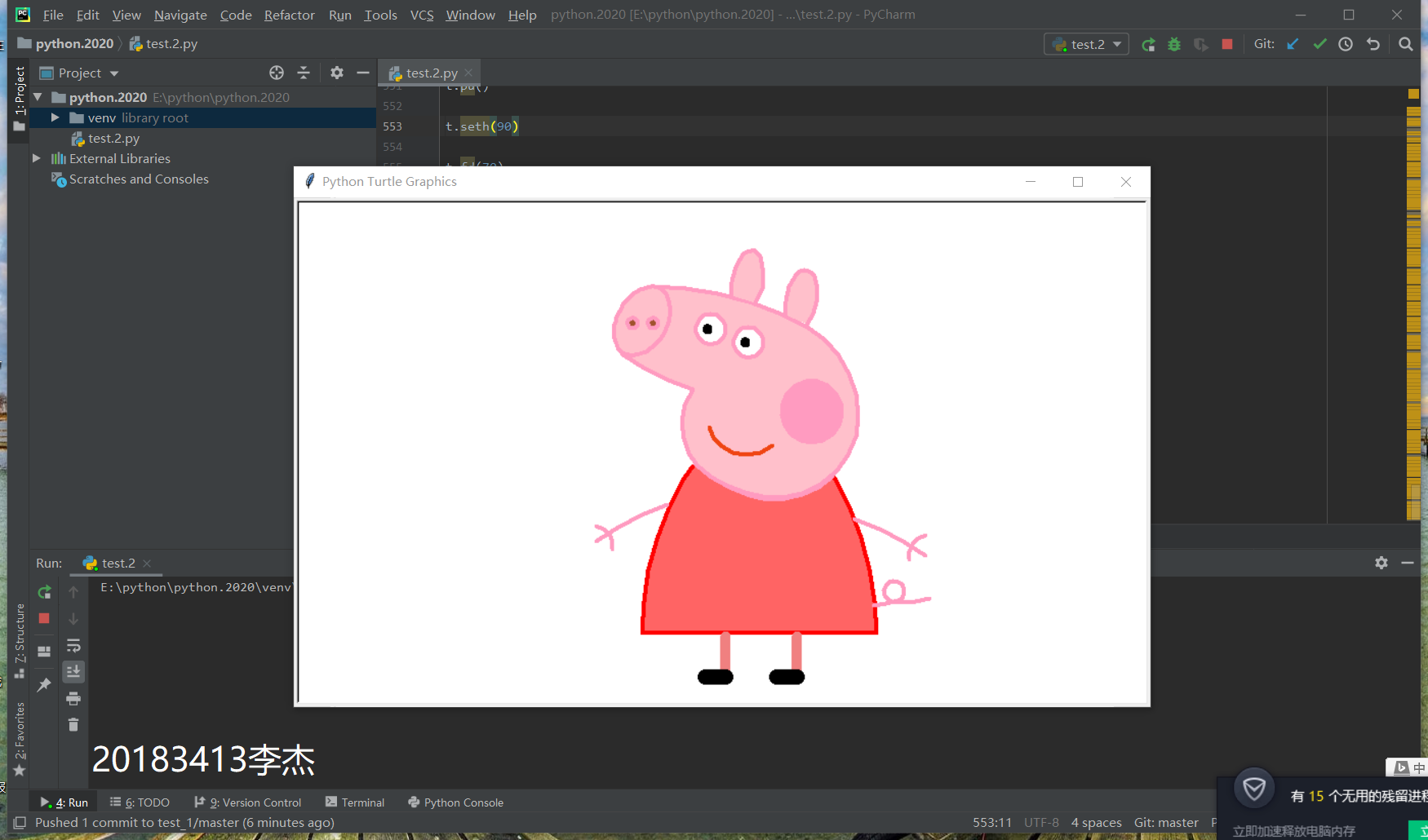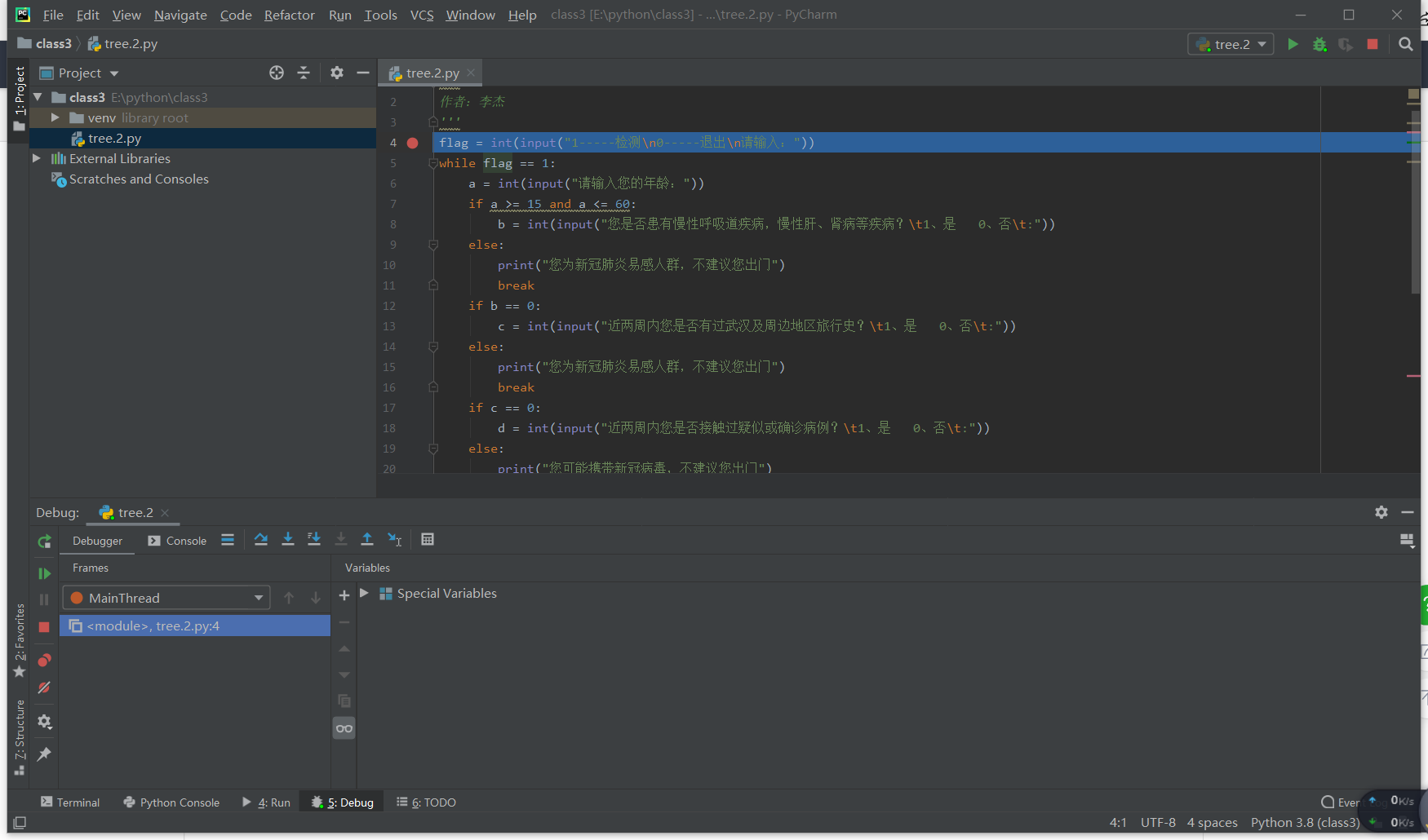### git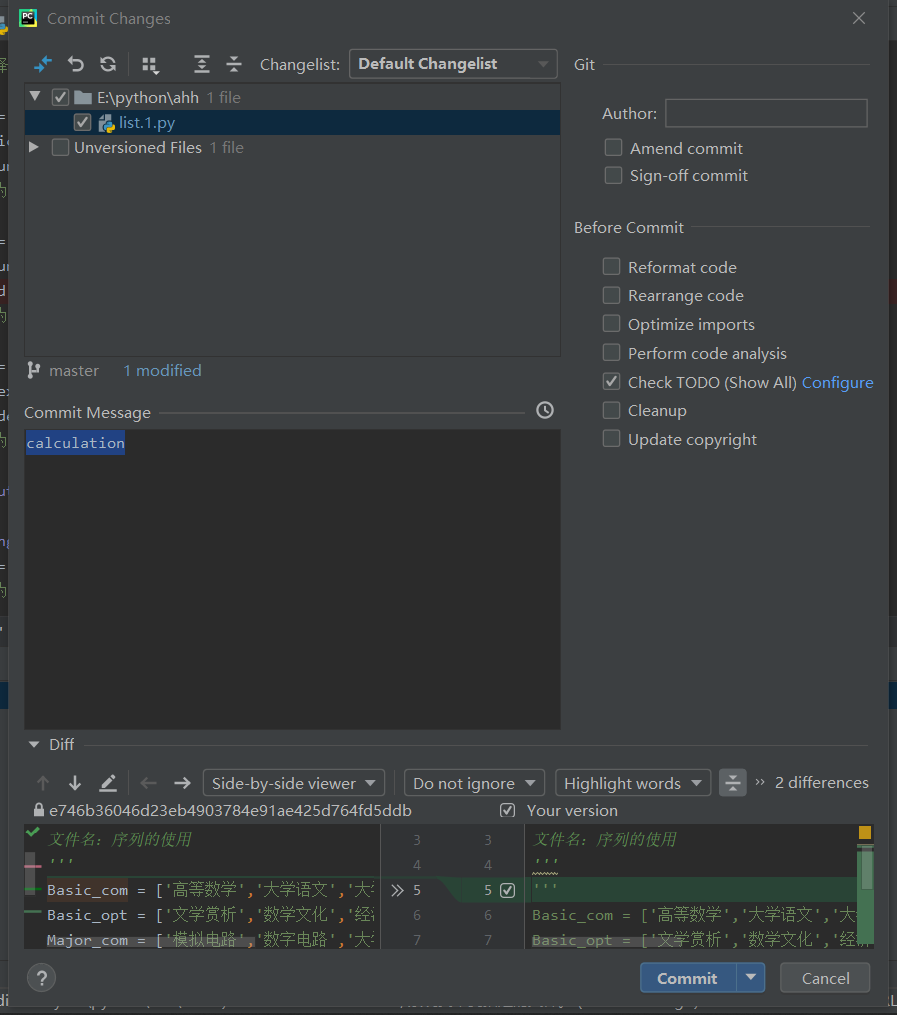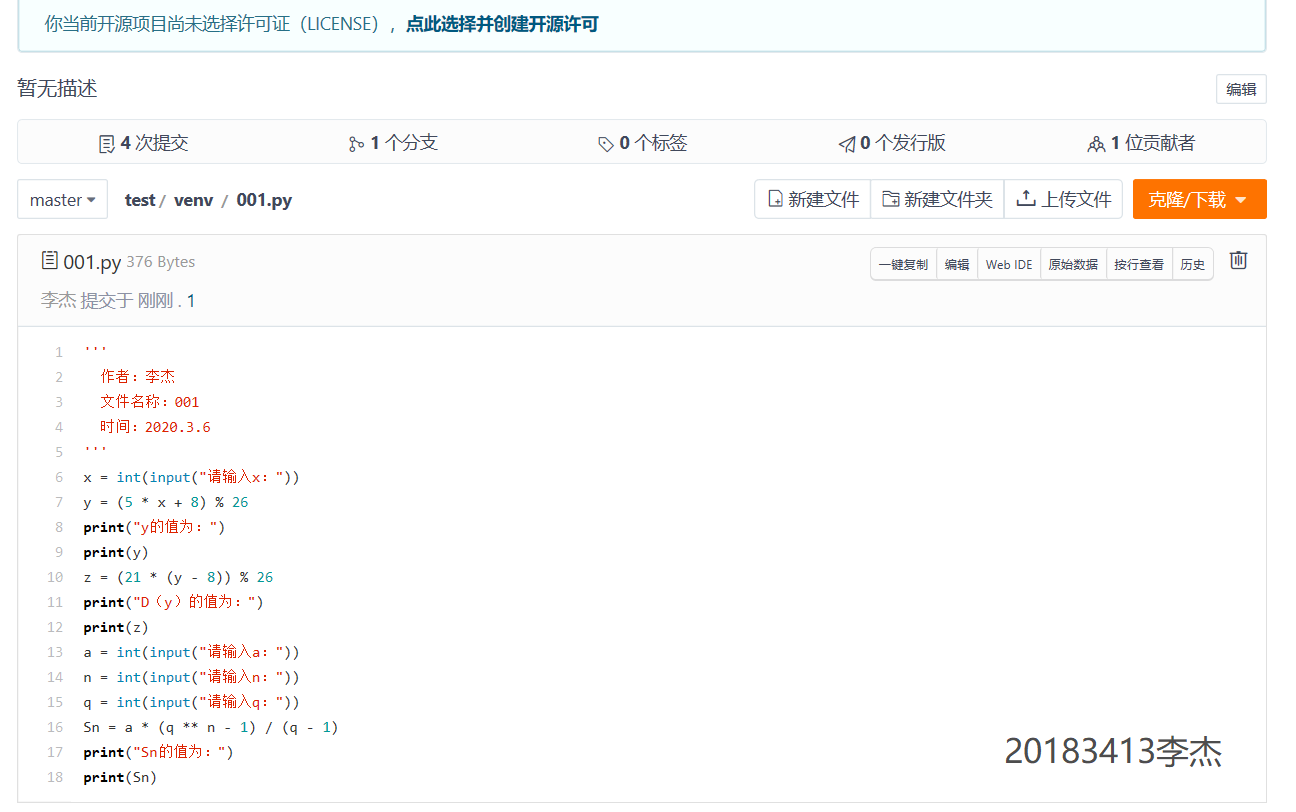## 3.实验过程中遇到的问题以及解决方案

• 问题1：设置断点后不能正常进行debug

问题1解决方案：上网查询原因，设置相关环境即可

• 问题2：误删某个文件后无法正常git

问题2解决方案：新建另一个project，在码云上新建一个仓库即可

## 其他

• python确实和我以前接触的C语言不一样，更加简便，但是pycharm的操作更加复杂，要多加练习才能够更好的掌握，相信学好了python以后自己的编程能力能够上一个台阶！

posted @ 2020-04-22 17:05  是老李啊  阅读(273)  评论(0编辑  收藏